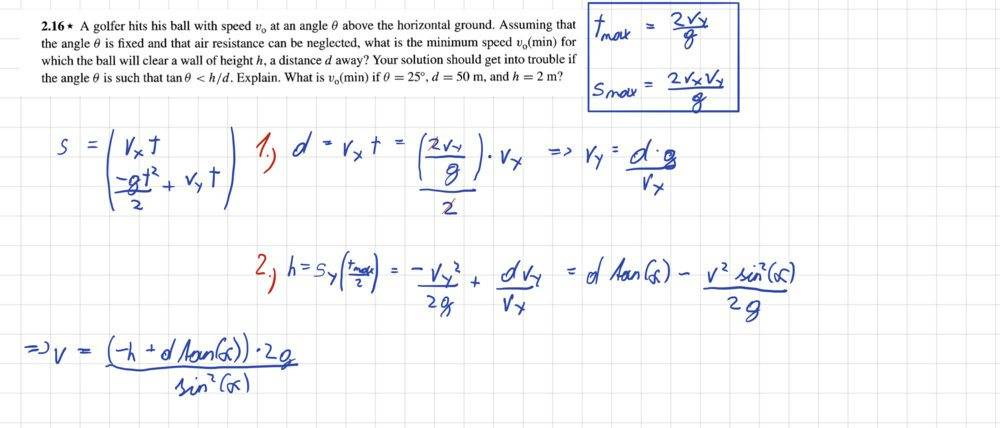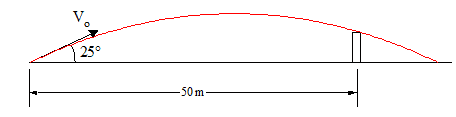# Clearing a wall with a golf shot

Oliver321
Homework Statement:
A golfer hits his ball with speed v at an angle phi above the horizontal ground. Assuming that the angle phi is fixed and that air resistance can be neglected, what is the minimum speed v(min) for which the ball will clear a wall of height h, a distanced away? Your solution should get into trouble if the angle is such that tan phi < h / d. Explain. What is v(min) if phi = 25°, d = 50 m, and h = 2 m?
Relevant Equations:
See picture below
Every trajectory follows a parabola if we neglect air resistance. So we can calculate the maximum distance in x direction s_max. Also we can determine the time it takes to hit the ground again t_max. If the ground is everywhere the same height, I can assume that at t_max/2 the height (s_y) is at a maximum. So at first i calculate the distance d at which the ball has its maximum height. I substituted this solution into s_y. Here I took the time t_max because the height has a maximum there. Now I could solve for v.

Nevertheless the solution is wrong (48 m/s). I know another way with which I get the right solution (26 m/s), but I don’t know what is wrong in my solution. Maybe you could help me!Last edited by a moderator:
•TSny

Homework Helper
Gold Member
You are assuming that the ball will be at its maximum height when it passes over the wall. Is this a correct assumption?

•Oliver321
Oliver321
You are assuming that the ball will be at its maximum height when it passes over the wall. Is this a correct assumption?

Thanks! I asked me this question also. But I came to the conclusion that this has to be. Because if the ball passes the wall before t_max/2, I could lower the velocitiy. So the peak would be closer to the origin and the height also decreases. But it could also be that I am wrong.

Mentor•Oliver321 and PeroK
•